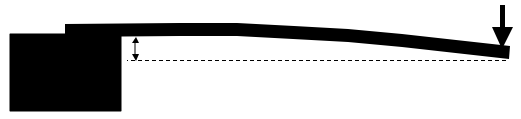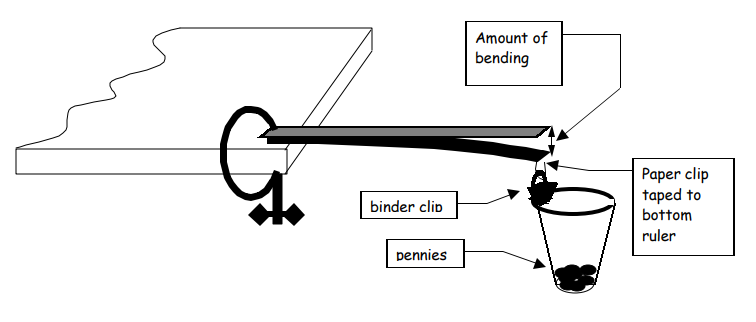Subject:
Mathematics

Topics: AlgebraPatternsLinear Equations and FunctionsPolynomial Equations and FunctionsFunctions and Relations
Common Core State Standard: 8.F.1, 8.F.2, 8.F.4, 8.F.5, F-IF.1, F-IF.2, F-IF.4,
Concepts:

· Function

Knowledge and Skills:

· Can explain the reasoning used to solve a problem
· Can plot a point in a two-dimensional coordinate system, given the coordinates, or determine the coordinates of a given point
· Can determine the equation of a linear function that closely matches a set of points (optional)
· Can determine the equation of a cubic function that closely matches a set of points (optional)

Materials:

(for each team)
· One “C” clamp
· plastic or strapping tape
· Two foot-long plastic rulers
· One ruler with millimeter markings
· One heavy duty paper clip
· One binder clip
· A plastic cup (the type with a thickened rim)
· 200 pennies

Lesson:

Procedure: This project should be done by students in teams of two to four.

Distribute the handout and review the first page with students, ensuring that they understand the term “cantilever.”

You may wish to discuss questions 1 and 2 as a class, or have the students answer them as part of the report they will prepare on their experiment. Discuss, if you wish, the balance of forces evidenced in diagram two, in which the downward force on the end of the cantilever must be equal to the upward force exerted by its support (otherwise it would be in motion).

Theoretically, students should find a linear relationship between the amount of weight (number of pennies) and the amount by which the cantilever (bottom ruler) bends. The amount of bending should vary as the cube of the length of the cantilever.

Depending on the level of the students, you may wish to have them determine the equations of the functions which most closely match the data, or simply identify them as “probably linear” and “probably not linear.”

Require the reports to be neatly organized and presented and clear in their explanations of the mathematical reasoning used.

### Cantilevers

A cantilever is simply a rigid plank of wood, a beam, or a pole which is fixed at one end.

An example is a diving board:When a force is applied to the free end, the cantilever will bend.1) Name as many variables as you can think of relating to the situation in diagram “B,” just above.

2) Describe how some of the variables you just named might be related to each other (for example, how do you think the “amount of bending” depends on the “amount of force applied”)?

3) Use materials as shown in the diagram below to investigate the situation in Diagram B, as follows:

a) Measure the amount of bending that occurs for different amounts of force applied. Draw a graph that represents your data. How are these two quantities related?

b) Do you think this is a function? Why or why not?

c) (Optional) Find an equation that is a good model for this relationship.

d) Measure the amount of bending that occurs for different cantilever lengths, with the same amount of force each time. Again, graph your data. How are these quantities related?

e) Do you think this is a function? Why or why not?

f) (Optional) Find an equation that is a good model for this relationship.

### Cantilever Investigation